• Submissions# Mathematical Analysis of Antibody by Gamma and PSI Functions

Bin Zhao*, Aibing Li and Lichun Liang

College of Science, Northwest A & F University, China

*Corresponding author: Bin Zhao, College of Science, Northwest A&F University, Yangling, Shaanxi, China

Submission: September 13, 2017; Published: December 18, 2017

DOI: 10.31031/APDV.2017.02.000535ISSN : 2576-9162
Volume2 Issue2

#### Abstract

This study aimed to develop mathematical models with important physiological parameters involving immune response for antibody by using Gamma and Psi functions, which could be used in many aspects, for instance to explain the controversies concerning antibody concentration with biological reasons and mathematical calculations, to optimize the antibody yield in large scale production. In this paper, we establish two new logarithmically completely monotonic functions involving the gamma function according to two preferred interaction geometries, necessary and sufficient conditions are presented for one of them to be logarithmically completely monotonic. As a consequence, a sharp inequality involving the gamma function is deduced to solve the problems of genetically engineered antibody. This approach would aid to utilize the engineered antibodies and their derivatives for various biomedical applications.

Keywords: Gamma function; Genetically engineered antibody; Logarithmically completely monotonic; Inequality; PSI function

#### Introduction

Mathematical or computational models have been proposed in different biological subjects in order to explain various complex phenomena. We have designed this study to monoclonal antibodies and Fc-fusion proteins (Figure 1). At present, it is still difficult to predict the optimal structure of antibodies. Topology knowledge can be important in antibody application as well as transformation. Theoretically, we can obtain desired antibodies by using protein/ gene engineering technology. For instance, we can transform the complementarities determining region (CDR) to promote the affinity of the antibody to antigen. Similarly, we could also transform any domain of antibody to make it bind with any desired target. Under this vision, topology is a powerful tool to predict the structure of protein and it will serve to antibody engineering. Our present work tries to explain, and predict, if possible, the change of structure, size and function of antibodies as well as their fragments from a topological perspective.

Figure 1: Main formats of monoclonal antibodies and Fc-fusion proteins licensed for use in rheumatology.
A. Diagram of infliximab (whole IgG1 molecule against TNFα). Red: VH and VL domains; Blue: constant domains of heavy and light chains. Therapeutic IgGs may differ regarding the heavy-chain isotype and the degree of variable domain humanization. For instance, ixekizumab is a humanized IgG4 and denosumab a fully human IgG2.
B. Etanercept is a fusion protein that combines the TNFα receptor to a human IgG1 Fc fragment. Dark red: cysteine-rich domains (CRD) of the TNFα receptor.
C. Certolizumab pegol is a pegylated Fab fragment. Green: polyethylene glycol chains. Glycosylated proteins (N-glycans and O-glycans) are not shown, to improve readability.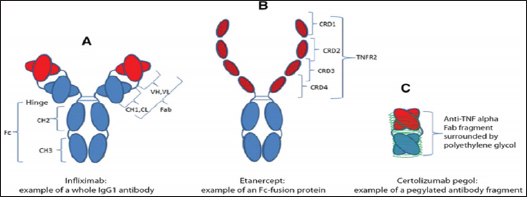Main formats of monoclonal antibodies and Fc-fusion proteins licensed for use in rheumatology (Figure 1).

a. Diagram of infliximab (whole IgG1 molecule against TNFα ). Red: VH and VL domains; Blue: constant domains of heavy and light chains. Therapeutic IgGs may differ regarding the heavy-chain iso type and the degree of variable domain humanization. For instance, Ixekizumab is a humanized IgG4 and denosumab a fully human IgG2.

b. Etanercept is a fusion protein that combines the TNF α receptor to a human IgG1 Fc fragment. Dark red: cysteine-rich domains (CRD) of the TNFα receptor.

c. Certolizumab pegol is a pegylated Fab fragment. Green: polyethylene glycol chains. Glycosylated proteins (N-glycans and O-glycans) are not shown, to improve readability.

For Re(z) > 0 the classical Euler's gamma function Г and psi (digamma) function Ψ are defined byrespectively. The derivatives Ψ(n)(z) for n ∈ ℕ are known as polygamma functions. For Ψ(z) , the following series representations are established: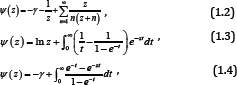Where, γ = 0.57721566490 --- denotes the Euler’s constant. We next recall [2-5] that a function f is said to be completely monotonic on an interval I, if f has derivatives of all orders on I which alternate successively in sign, that is,

(-1 )nf(n) (x) ≥ 0 , (1.5)

for all x ∈ I and for all n ≥ o . If inequality (1.5) is strict for all x ∈ I and all n ≥ o , then f is said to be strictly completely monotonic. The classical Bernstein-Widder theorem , Theorem 12a states that a function f is completely monotonic on (o, ∞) if and only if it is a Laplace transform of some nonnegative measure μ that is,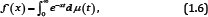Where, μ(t) is non-decreasing and the integral converges for x > o . We recall also [7-9] that a positive function f is said to be logarithmically completely monotonic on an interval I if f has derivatives of all orders on I and

(-1)n[lnf(x)](n)≥0 , (1.7)

for all xI and for all n ≥ 1 . If inequality (1.7) is strict for all x ∈ I and all n ≥ 1, then f is said to be strictly logarithmically completely monotonic. The antibody structure will be changed when it binds certain target (Figure 2), i.e.: antigen, receptor. How to describe the changes in the view of topology? The following cases will explain it in detail.

Diagrammatic representation of VH germ-line diversity (top) leading to domain structure diversity (bottom) (Figure 2). In humans, multiple V, D, J regions are rearranged to produce a VDJ segment that encodes the variable region (in this case the VH). The assembled polypeptide is represented by the classically ‘Y’ shaped IgG (150kDa) molecule (bottom), where the various regions of the antibody are labeled and the carbohydrates are indicated by pink dots. Unlike humans or mice, chickens use gene conversion to diversify the antibody repertoire. Single genes encode the VH (and VL) and a single VDJ event, which generates minimal diversity, is preceded by insertion of upstream pseudogenes (WH] in a process that results in donation of diverse sequence fragments into the V region. Chicken antibody (IgY) has a molecular weight of 180 kDa by virtue of an additional constant heavy domain and additional carbohydrate moieties. Cows employ an ultra long CDRH3 repertoire resulting from a VDJ event that utilises a single VH segment (VH Bul), which combines with an elongated D region (DH2) to produce a recombined V-region that undergoes diversification by somatic hyper-mutation. This can introduce or remove cysteine thus, diversifying the disulfide landscape of the repertoire.

Figure 2: Diagrammatic representation of VH germ-line diversity (top) leading to domain structure diversity (bottom).It was proved explicitly in  and other articles that a logarithmically completely monotonic function must be completely monotonic. In , G. D. Anderson et al. proved that the function

g (x) = x(ln x-Ψ(x)) (1.8)

is strictly decreasing and strictly convex on (o, ∞) , with two limitsFrom (1.9) and the monotonicity of g (x), then the double inequalities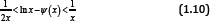holds for all x > o .

In , Theorem , by using the well-known Binet's formula, H. Alzer generalized the monotonicity and convexity of s (x) , that is, the function

gα(x) = -xα (ln x-Ψ(x)) (1.11)

is strictly completely monotonic on (o, ∞) if and only if α≤1.

In , D. Kershaw and A. Laforgia proved that the function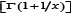x is decreasing on (o, ∞) and xx is increasing on (0,∞) . These are equivalent to the function1/xbeing increasing and1/x/x being decreasing on (o, ∞) , respectively. In Theorem 5, F Qi & Ch-p Chen  generalized these functions. They obtained the fact that for all x > o the function xr1/x is strictly increasing for r ≥ o and strictly decreasing for r ≤ -1, respectively. After the papain digestion, the remained antibody functional part (usually the Fab domain), will be smaller and the structure is also changed. These changes can be revealed vividly using topology. Recently [14,15], Theorem 1, F Qi, et al.  established another excellent result, which states that forgiven y ∈ (-1, ∞) and α ∈ (-∞, ∞), letThe function (1.12) is logarithmically completely monotonic with respect to x∈(-y-1 ∞) if and only if α≥ max {1,1/ (y +1)}; and if α ≤ min {1,1/2 (y + 1)}, the reciprocal of the function (1.12) is logarithmically completely monotonic with respect to x ∈ (— y — 1,∞) . Antibodies occur spontaneously gathering and forming dimer, polymer, which will influence their functions (Figure 3). In antibody engineering practice, it urgently needs some measures to overcome this difficulty. From topology perspective, we could understand this issue as follow. Stimulated by the above results, we put forward the function as follows: forgiven y ∈ (o, ∞) and real number α, let the function fαy (x) be defined by#### Our First Result is Contained in the Following Theorem

Theorem 1: For the function (1.13), then the following statements are true:

i. For any given y ≥ 1 , the function (1.13) is strictly logarithmically completely monotonic with respect to x∈(— y,∞) \ {o} if and only if α≥ 1;

ii. For any given o <y< l, if α ≥ e-(1-y) Y , then the function (1.13) is strictly logarithmically completely monotonic with respect to x∈(— y,∞) \ {o}

iii. For any given y > o, the reciprocal of the function (1.13) is strictly logarithmically completely monotonic with respect to x∈(— y,∞) \ {o} if and only if α < o .

Our second result is presented in the following theorem.

Theorem 2: For any given y ∈ [1,∞), let the function hy (x) be defined on (o, ∞) byWhere, γ denotes the Euler’s constant, then the function (1.14) is strictly logarithmically completely monotonic with respect to x on (o,∞) . The following corollary can be derived from Theorems 2 immediately.

Corollary 1: For any given y ≥ 1, the inequality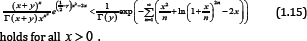#### Lemma

In order to prove our main results, we need the following lemmas. It is well known that Bernoulli polynomials Bk (x) and Euler polynomials Ek (x) are defined byrespectively. The Bernoulli numbers Bn are denoted by Bn = Bn (o), while the Euler numbers En are defined by . In , the following summation formula is given:The Bernoulli and Euler numbers and polynomials are generalized [18-21].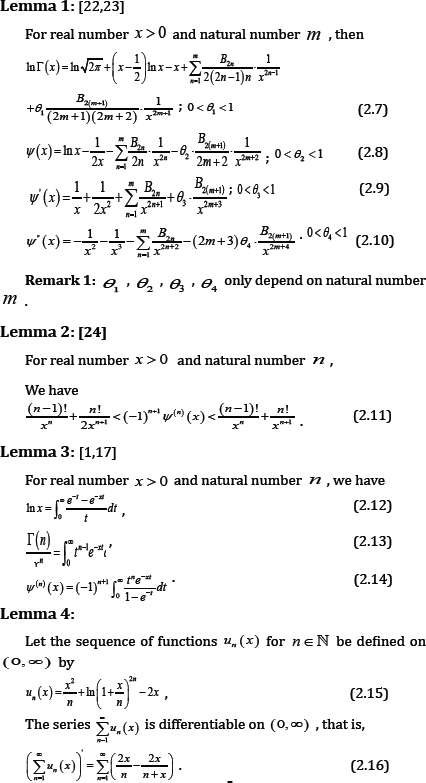Proof: It is obvious that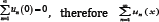converges at x = 0. In order to prove (2.16), we need only to show that the inner closed uniform convergence of the serieson (0,∞) . From (2.15), We have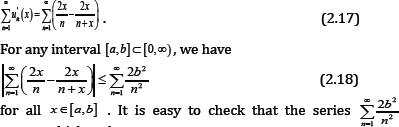converges, which and

Weierstrass M-test implies that the seriesis inner closed uniformly convergent on (0, ∞). Hence, the seriesis differentiable on (0, ∞) and the identity (2.17) holds for x ≥ 0 .

The lemma is proved.

### Lemma5:

For 0 < α ≤ 1 and real number b , let the function Qa,b (x) be defined bya. Case 1: If O< α≤ 1≤b ,then since q (t) > 0 for t > 0, we haveWhich, implies αt <(l + bt)(l-e -t), and then p (t )> 0 for all t > 0 .

From (2.24), we know that the inequality (2.20) holds for x ∈(-b/ α, ) and integer n ≥ 2.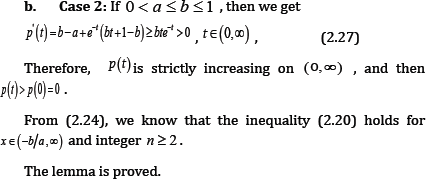### Proof of Theorem 1

For x ≠ 0 and natural number n , taking the logarithmically differential into consideration yields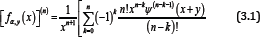Where, Ψ(—1)(x + y) and Ψ(0)y) and Ψ( x+y) respectively.

Furthermore, differentiating xn+1 [Fαy(x)](n)directly givesMaking use of (2.11) and (2.13) shows that for all n∈ℕ and any fixed y > o, the double inequalityOn the other hand, if x∈(0,∞) then the inequalities (3.10) can be equivalentlyHence, if either α≥ e-(1-y)/y for given o < y < 1 or α≥ 1 for given y ≥ 1, the function (1.13) is strictly logarithmically completely monotonic with respect to x on (o,⊂), and if α≤o forgiven y>o, so is the reciprocal of the function (1.13).

On the other hand, if x∈(-y,0) for any given y > o, then (3.10) impliesfor n ∈ℕ . It is obvious that (3.18) is equivalent to that (3.14) and (3.15) hold for any given y > o and x∈(-y,0). Therefore, it is easy to prove similarly that (3.16) is also valid on x∈(-y,0) for any given y > o and all n ∈ℕ .

Figure 3: Unique Structural Domain in Bovine IgG antibodies and application.The amino acid of antibody/protein possesses different preferences. Thus we can conduct site-directed mutation to promote the affinity and/or hydrophilic with the prediction of topology. For example, bovine antibodies have an unusual structure comprising a β-strand 'stalk' domain and a disulphide-bonded 'knob' domain in CDR3 (Figure 3). Attempts have been made to utilize such amino acid preference for antibody drug development. Unique Structural Domain in Bovine IgG antibodies and application (Figure 3).

Consequently, the function (1.13) is the same logarithmically completely monotonicity on (-y,0) as on (0,∞), that is, if either α≥e-(1-y)/y for given 0<y<1 or α≥ 1 for given y1 , the function (1.13) is strictly logarithmically completely monotonic with respect to x on (-y,0) , and if α≤0 forgiven y > 0, so is the reciprocal of the function (1.13).

Conversely, we assume that the reciprocal of the function (1.13) is strictly logarithmically completely monotonic on (-y,∞)\{0} for any given y > 0. Then we have for any given y > 0 and all x > 0for any given y > 0. By virtue of (3.20) and (3.21), we conclude that the necessary condition for the reciprocal of the function (1.13) to be strictly logarithmically completely monotonic is α≤ 0.

If the function (1.13) is logarithmically completely monotonic on (-y,∞)\ {0} for any given y > 0 , then the inequality (3.19) and (3.20) are reversed for any given y > 0 and all x > 0.for any given y > 0 . In fact, it is not difficult to show that the necessary condition for the function (1.13) to be strictly logarithmically completely monotonic is α ≥ 1. The proof of Theorem 1 is completed.

### Proof of Theorem 2By Lemma 5, we know that μ' (x) is strictly increasing on (0,∞ ), which and (1.10) imply the limit of μ'(x) equals 1 as x →∞, therefore

μ'( x)< 1 (3.29)

holds for all x > 0 .

We know that g (x) is strictly completely monotonic on (0,∞), where g (x) is defined by (1.8), hence for given integer n ≥ 0, the inequality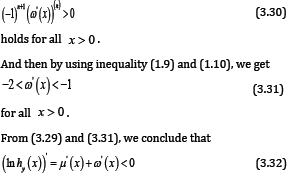for all x > 0 . Utilizing Lemma 5 and (3.30), for given integer n ≥ 2, it is easy to see that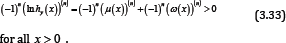for all x > 0 .

Theorem 2 follows from (3.32) and (3.33). Thus the proof of Theorem 2 is completed [25-31].

#### Conclusion

In conclusion we establish two new logarithmically completely monotonic functions involving the gamma function according to two preferred interaction geometries, and a sharp inequality involving the gamma function is deduced to solve the problems of genetically engineering antibody. It is necessary to address, many other aspects (such as thermal condition, alkalinity or acidity, adhesion of antibody) are also playing key roles in antibody functioning, which could be also understood from bio-mathematical perspective, and such knowledge will be in return useful for biomedical application of antibodies as well as proteins.

#### Acknowledgement

This work was supported by the Fundamental Research Funds for the Central Universities (2014YB030), Ministry of Education and State Administration of Foreign Experts Affairs "Overseas Teacher" project (MS2011XBNL057), the Key Construction Pro- gram (2015SD0018) of International Cooperation Base in S&T, Shaanxi Province, China.

© 2017 Bin Zhao, et al. This is an open access article distributed under the terms of the Creative Commons Attribution License , which permits unrestricted use, distribution, and build upon your work non-commercially.

We at Crimson Publishing are a group of people with a combined passion for science and research, who wants to bring to the world a unified platform where all scientific know-how is available read more...